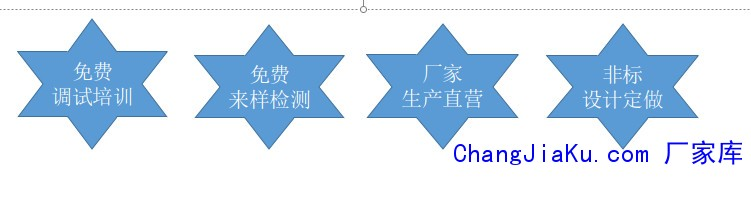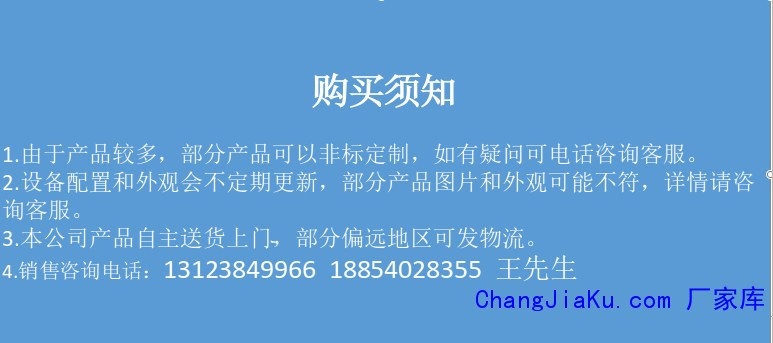客服热线：133-4342-757513123849966•••# 德迅 电子拉力试验机DWAW-300KN 液压式拉力试验机 压力试验机 剥离试验机 金属 塑料等多种材料试验机

• 52000.00元/台

CJK-1593

13123849966 057456215827
• 发货地  浙江
• 供货总量  99 台

• 暂无产品目录
 有效期至长期有效 更新时间2020-05-09 10:36 访问次数667

rr

r

r
r

r

r
r

r

r 主机为四立柱、两丝杠、油缸下置式，拉伸空间位于主机的上方，压缩、弯曲试验空间位于主机下横梁和工作台之间。测控系统采用全数字多通道闭环测控系统，具有三闭环功能，即可以进行应力、应变、位移闭环等控制方式，并可以进行无冲击切换，其应力速度和应变速率完全符合国标GB/T228.1-2010等其它金属拉伸试验标准要求。r

r

r
r

r

r
r

r

r 二、 传动系统r

r

r 下横梁升降采用电机经减速器、链传动机构、丝杠副传动，实现拉伸、压缩空间的调整。r

r

r 三、 液压系统r

r

r r

r
r

r
r

r

r 油箱内的液压油通过电机带动高压泵进入油路，流经高压滤油器、压差阀组、伺服阀，进入油缸。计算机发出控制信号到伺服阀，控制伺服阀的开口和方向，从而控制进入油缸的流量，实现等速试验力、等速位移等的控制。r

r

r 四、 控制系统r

r

r l 支持进行拉伸、压缩、剪切、弯曲等试验；r

r

r l 支持开放编辑试验、编辑标准和编辑步骤，并支持导出导入试验、标准和步骤；r

r

r l 支持试验参数自定义；r

r

r l 采用开放式的EXCEL报表形式，支持用户自定义报表格式；r

r

r l 查询打印试验结果灵活方便，支持打印多个试样，自定义排序打印项目；r

r

r l 程序自带强大试验分析功能；r

r

r l 程序支持分级管理两级（管理员、试验员）用户管理权限；r

r

r 五、软件描述r

r

r （1）软件可以在Windows98/2000/XP，用户界面呈现与Windows风格一致的中文窗口系统。r

r

r
r

r

r (2)自动程控可选用多种控制模式。r

r

r
r

r

r
r

r

r （3）自动程控智能专家系统。自动程控步骤可达50个步骤。r

r

r
r

r

r （4）报表编辑：r

r

r
r

r

r (5) 试验方法种类多,可选择r

r

r （6）软件设有三级管理权限，分别由各自的口令登录，进一步保证了软件安全使用。r

r

r
r

r

r 六、安全保护装置r

r

r 当试验力超过最大试验力的3%时，过载保护，油泵电机停机。r

r

r 当活塞升起达到上极限位置时，限位保护，油泵电机停机。r

r

r 当横梁下降达到设定位置时，限位保护，减速机停机。r

r

r 七、 主要性能技术指标r

rr r r r r r r r r r r r r r r r r r r r r r r r r r r r r r r r r r r r r r r r r r r r r r r r r r r r r r r r r r r r r r r r r r r r r r r r r r r r r r r r r r r r r r r r r r r r r r r r r r r r r r r r r r r r r
 r r 最大试验力（kN） r r r r 300 r r r r   r r r r 试验力测量范围 r r r r r r r r 全程不分档，等效4档 r r r r 试验力示值相对误差 r r r r ＜ 示值的±0.5% r r r r   r r r r 试验力分辨力 r r r r 最大载荷1/300000 r r r r   r r r r 变形示值相对误差 r r r r ＜ 示值的±0.5% r r r r   r r r r 变形分辨力 r r r r 最大变形量1/300000 r r r r   r r r r 最大拉伸试验空间（mm） r r r r 600 r r r r   r r r r 压缩空间（mm） r r r r 430 r r r r   r r r r 活塞行程(mm) r r r r 200 r r r r   r r r r 立柱间距（mm） r r r r 480 r r r r   r r r r 圆试样夹持范围（mm） r r r r Φ6-Φ40 r r r r   r r r r 扁试样夹持厚度（mm） r r r r 0-15 r r r r   r r r r 扁试样最大夹持宽度（mm） r r r r 75 r r r r   r r r r 压盘尺寸（mm） r r r r φ160mm r r r r   r r r r 弯曲支点两点间最大距离（mm） r r r r 300 r r r r   r r r r 弯曲支滚宽度（mm） r r r r 140 r r r r   r r r r 夹紧方式 r r r r 液压夹紧 r r r r   r r r r 主机外形尺寸（mm） r r r r 760*620*2200 r r r r   r r r r 油源控制台尺寸（mm） r r r r 1100*750*1000 r r r r   r r r r 电机总功率（kW） r r r r 2 r r r r   r r r r 总重量（kg） r r r r 2600 r r r r   r r
rr

r 八、出厂标准配置清单r

rr r r r r r r r r r r r r r r r r r r r r r r r r r r r r r r r r r r r r r r r r r r r r r r r r r r r r r r r r r r r r r r
 r r 试验机主机 r r r r 四立柱、两丝杠、油缸下置式 r r r r 1台 r r r r 压力力传感器 r r r r 美国世铨精密传感器 r r r r 1只 r r r r 伺服控制油源 r r r r 专有技术 r r r r 1台 r r r r 软件控制系统 r r r r 含软件、位移传感器 r r r r 1套 r r r r 电器控制系统 r r r r 专有技术 r r r r 1套 r r r r 计算机 r r r r 联想商用品牌电脑 r r r r 1台 r r r r 打印机 r r r r 喷墨式打印机 r r r r 1台 r r r r 圆试样拉伸辅具 r r r r Φ13-Φ26、Φ26-Φ40 r r r r 各1套 r r r r 扁试样拉伸辅具 r r r r 0-15mm r r r r 1套 r r r r 压缩辅具 r r r r φ160mm r r r r 1套 r r r r 电子引伸计 r r r r 刚研所 r r r r 一套 r r r r 说明书合格证装箱单 r r r r ----------- r r r r 1套 r r
r

r
r

r

r
r

## 德迅 电子拉力试验机DX-200KN 拉力试验机r rrrrrrrrr使用领域：rrr该产品广泛用于金属、非金属材料的拉、压、弯剪等力学性能试验。配以种类繁多的附具，还可用于型材和构件的力学性能试验。在试样变形大，试验速度快的绳、带、丝、橡胶、塑料等材料试验领域，同样具有非常广泛的应用前景。适用于质量监督、教学科研、航空航天、钢铁冶金、汽车、建工建材等试验领域。rrr依据标准：rrr满足标准GB/T228.1-2010《金属材料室温拉伸试验方法》、GB/T7314-2005《金属压缩试验方

## 德迅 电子拉力试验机DX-50KN 拉力试验机 压## 疲劳试验机 电液伺服动静疲劳试验机 5Hz 10***************************************************************************************************************************************************************************************************************************************************************

## 电子拉力试验机 环刚度测试 材料试验机## 德迅DX-20KN 电子拉力试验机 拉力试验机 剥## 德迅 WAW-600KN 拉力试验机 压力试验机 剥## 德迅DX-10KN 电子拉力试验机 拉力试验机 拉r rrrrrrrrr使用领域：rrr该产品广泛用于金属、非金属材料的拉、压、弯剪等力学性能试验。配以种类繁多的附具，还可用于型材和构件的力学性能试验。在试样变形大，试验速度快的绳、带、丝、橡胶、塑料等材料试验领域，同样具有非常广泛的应用前景。适用于质量监督、教学科研、航空航天、钢铁冶金、汽车、建工建材等试验领域。rrr依据标准：rrr满足标准GB/T228.1-2010《金属材料室温拉伸试验方法》、GB/T7314-2005《金属压缩试验方

## 德迅 电子拉力试验机DX-100KN 拉力试验机## 数显扭力试验机 金属扭力试验机 弹簧扭力试## 电子拉力试验机DX-200KN 拉力试验机 压力试